## Example Questions

### Example Question #1 : Decimals With Fractions

If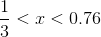can equal which of the following?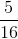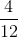None of the other answer choices are correctExplanation:

Convert all of the fractions to decimals. Thus, x is contained within the range of 0.33 < x < 0.76. The answers choices become 1/4 = 0.25, 4/12 = 0.33, 2/5 = 0.4, and 5/16 = 0.3125, respectively.  Therefore, the only answer which is within the desired range is 2/5.

### Example Question #1 : How To Find The Decimal Equivalent Of A Fraction

Vega only purchases gardening claws in bulk cases. Each case is 150 claws for $2415.17. Quantity A: The number of claws Vega can purchase with$41,082.05

Quantity B: The number of claws Vega can purchase with $43,473.05 Possible Answers: Quantity B is greater. Quantity A is greater. The two quantities are equal. The relationship cannot be determined. Correct answer: The two quantities are equal. Explanation: Looking at the differences in prices, one might guess that Quantity B is the answer. Beware the easy answer; always check. Instead, calculating how many cases of claws Vega can buy, keeping in mind that he cannot purchase part of a case. Do not round up! Quantity A: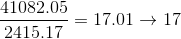Quantity B: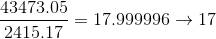In either case, he can buy no more than seventeen cases. The two quantities are equal. ### Example Question #2 : How To Find The Decimal Equivalent Of A Fraction Isley only buys seeds for her gardening store in bulk cases, with each case containingpackets of seeds and costing$343.25.

Quantity A: The number of seeds Isley can purchase with $3837.54 Quantity B: The number of seeds Isley can purchase with$3871.86

The relationship cannot be determined.

Quantity A is greater.

Quantity B is greater.

The two quantities are equal.

The two quantities are equal.

Explanation:

Looking at how close the dollar values are for A and B, it's tempting to think the GRE is being tricky, and that B will allow for the purchase of more cases of seeds.

Do not assume; check to see whether or not this is the situation. Note that a portion of a case cannot be purchased; round down to the nearest integer when calculating how many cases may be bought.

Since each case is \$343.25

Quantity A: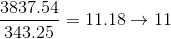Quantity B: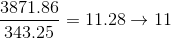The two quantities are equal.

### Example Question #3 : How To Find The Decimal Equivalent Of A Fraction

Choose the answer which best expresses the following fraction in decimal form (round to the nearest thousandth):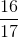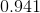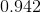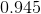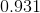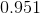Explanation:

To convert a fraction into a decimal, you simply need to divide the numerator by the denominator.  When the problem prompts you to round to the nearest thousandth, that means that you round to the third decimal place (i.e. go to the third decimal place, if the fourth is four or lower, you can leave the third decimal place digit alone, if the fourth is five or higher, raise the third decimal place digit by one).

When you divide 16 by 17 you will need to add a zero and a decimal place to 16 making it 160. From here we see that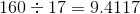but since we added a decimal place to 16 to create 160 we now need to move the decimal place of the answer one unit to the left.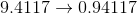Tired of practice problems?

Try live online GRE prep today.